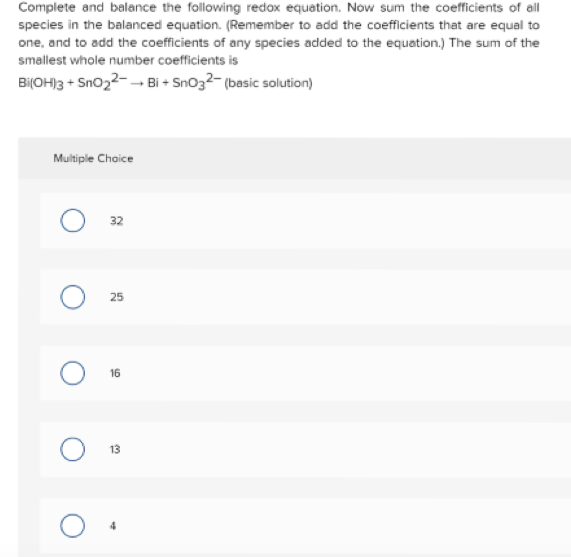# Complete and balance the following redox equation. Now sum the coefficients of all species in the balanced equation_ (Remember to add the coefficients that are equal to one_ and to add the coefficients of any species added to the equation.) The sum of the smallest whole number coefficients is Bi(0H)3 + Sn02^2-— Bi + Sn03^2-— (basic solution) 32 25 16 13 4